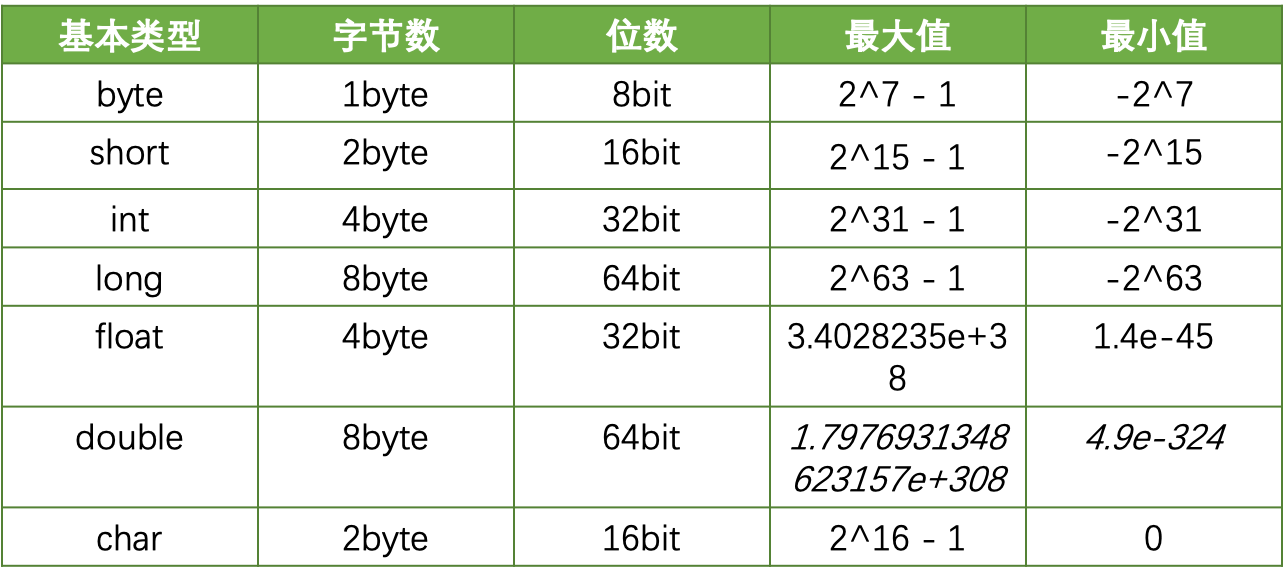# 简介

java中可以被称为Number的有byte，short，int，long，float，double和char，我们在使用这些Nubmer的过程中，需要注意些什么内容呢？一起来看看吧。

# Number的范围``````    public void testIntegerOverflow(){
System.out.println(Integer.MAX_VALUE+1000);
}
``````

• 第一种方式：在做Integer操作之前，进行预判断是否超出范围：

``````    static final int safeAdd(int left, int right) {
if (right > 0 ? left > Integer.MAX_VALUE - right
: left < Integer.MIN_VALUE - right) {
throw new ArithmeticException("Integer overflow");
}
return left + right;
}
``````

``````    public static int addExact(int x, int y) {
int r = x + y;
// HD 2-12 Overflow iff both arguments have the opposite sign of the result
if (((x ^ r) & (y ^ r)) < 0) {
throw new ArithmeticException("integer overflow");
}
return r;
}
``````

``````    public int addUseMath(int a, int b){
}
``````
• 第三种方式：向上转型

``````    public static long intRangeCheck(long value) {
if ((value < Integer.MIN_VALUE) || (value > Integer.MAX_VALUE)) {
throw new ArithmeticException("Integer overflow");
}
return value;
}

public int addUseUpcasting(int a, int b){
return (int)intRangeCheck((long)a+(long)b);
}
``````

• 第四种方式：使用BigInteger

``````    public int useBigInteger(int a, int b){
}
``````

# 区分位运算和算数运算

``````x += (x << 1) + 1;
``````

``````    public void testBitwiseOperation(){
int i = -10;
System.out.println(i>>>2);
System.out.println(i>>2);
System.out.println(i/4);
}
``````

# 兼容C++的无符号整数类型

``````    public int readIntWrong(DataInputStream is) throws IOException {
}
``````

``````    public long readIntRight(DataInputStream is) throws IOException{
}
``````

# NAN和INFINITY

``````public static final double POSITIVE_INFINITY = 1.0 / 0.0;
public static final double NEGATIVE_INFINITY = -1.0 / 0.0;
public static final double NaN = 0.0d / 0.0;
``````
``````public static final float POSITIVE_INFINITY = 1.0f / 0.0f;
public static final float NEGATIVE_INFINITY = -1.0f / 0.0f;
public static final float NaN = 0.0f / 0.0f;
``````

1除以0就是INFINITY，而0除以0就是NaN。

``````public void compareInfinity(){
System.out.println(Double.POSITIVE_INFINITY == Double.POSITIVE_INFINITY);
}
``````

``````    public void compareNaN(){
System.out.println(Double.NaN == Double.NaN);
}
``````

``````System.out.println(Double.isNaN(Double.NaN));
``````

``````    public void incorrectParse(String userInput){
double val = 0;
try {
val = Double.valueOf(userInput);
} catch (NumberFormatException e) {
}
//do something for val
}
``````

``````    public void testNaN(){
System.out.println(Double.valueOf("NaN"));
System.out.println(Double.valueOf("Infinity"));
System.out.println(Double.valueOf("-Infinity"));
}
``````

``````NaN
Infinity
-Infinity
``````

``````public void correctParse(String userInput){
double val = 0;
try {
val = Double.valueOf(userInput);
} catch (NumberFormatException e) {
}
if (Double.isInfinite(val)){
// Handle infinity error
}

if (Double.isNaN(val)) {
// Handle NaN error
}
//do something for val
}
``````

# 不要使用float或者double作为循环的计数器

``````for (float x = 0.1f; x <= 1.0f; x += 0.1f) {
System.out.println(x);
}
``````

0.6转成为二进制格式是乘2取整，0.6×2=1.2，取整剩余0.2，继续上面的步骤0.2×2=0.4，0.4×2=0.8,0.8×2=1.6,取整剩余0.6，产生了一个循环。

# BigDecimal的构建

``````    public void getFromFloat(){
System.out.println(new BigDecimal(0.1));
}
``````

``````    public void getFromString(){
System.out.println(new BigDecimal("0.1"));
}
``````

# 类型转换问题

• short to byte or char
• char to byte or short
• int to byte, short, or char
• long to byte, short, char, or int
• float to byte, short, char, int, or long
• double to byte, short, char, int, long, or float

• byte to short, int, long, float, or double
• short to int, long, float, or double
• char to int, long, float, or double
• int to long, float, or double
• long to float or double
• float to double

``````    public void intToByte(int i){
if ((i < Byte.MIN_VALUE) || (i > Byte.MAX_VALUE)) {
throw new ArithmeticException("Value is out of range");
}
byte b = (byte) i;
}
``````

``````    public void intToFloat(){
System.out.println(subtraction(1111111111,1111111111));
}

public int subtraction(int i , float j){
return i - (int)j;
}
``````

``````    public int subtraction(int i , float j){
System.out.println(j);
if ((j > 0x007fffff) || (j < -0x800000)) {
throw new ArithmeticException("Insufficient precision");
}
return i - (int)j;
}
``````

``````    public int subtractionWithDouble(int i , double j){
System.out.println(j);
return i - (int)j;
}
``````

learn-java-base-9-to-20/tree/master/security